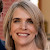# [untitled worksheet]

Crossword

Kindly Shared By:United States of America

Date Shared: 16 December 2019

Worksheet Type:

Tags Describing Content or Audience:

Worksheet Instructions:

None provided.

Target Language or Knowledge:

Translation A transformation that slides a figure from one position to another without turning. Transformation An operation that maps a geometric figure, pre-image, onto a new figure, image. Reflection A transformation where a figure is flipped over a line. Also called a flip. Rotation A transformation in which a figure is turned about a fixed point. Dilation A transformation that enlarges or reduces a figure by a scale factor. y axis The vertical line that divides the center of the plane x axis The horizontal line that divides the center of the plane Ordered pair A pair of numbers used to locate a point in the coordinate plane. (x,y) Congruent Figures have the same shape and same size. Quadrants The four sections of the coordinate plane.

#### Discussion Be the first to comment about this worksheet.16 December 2019